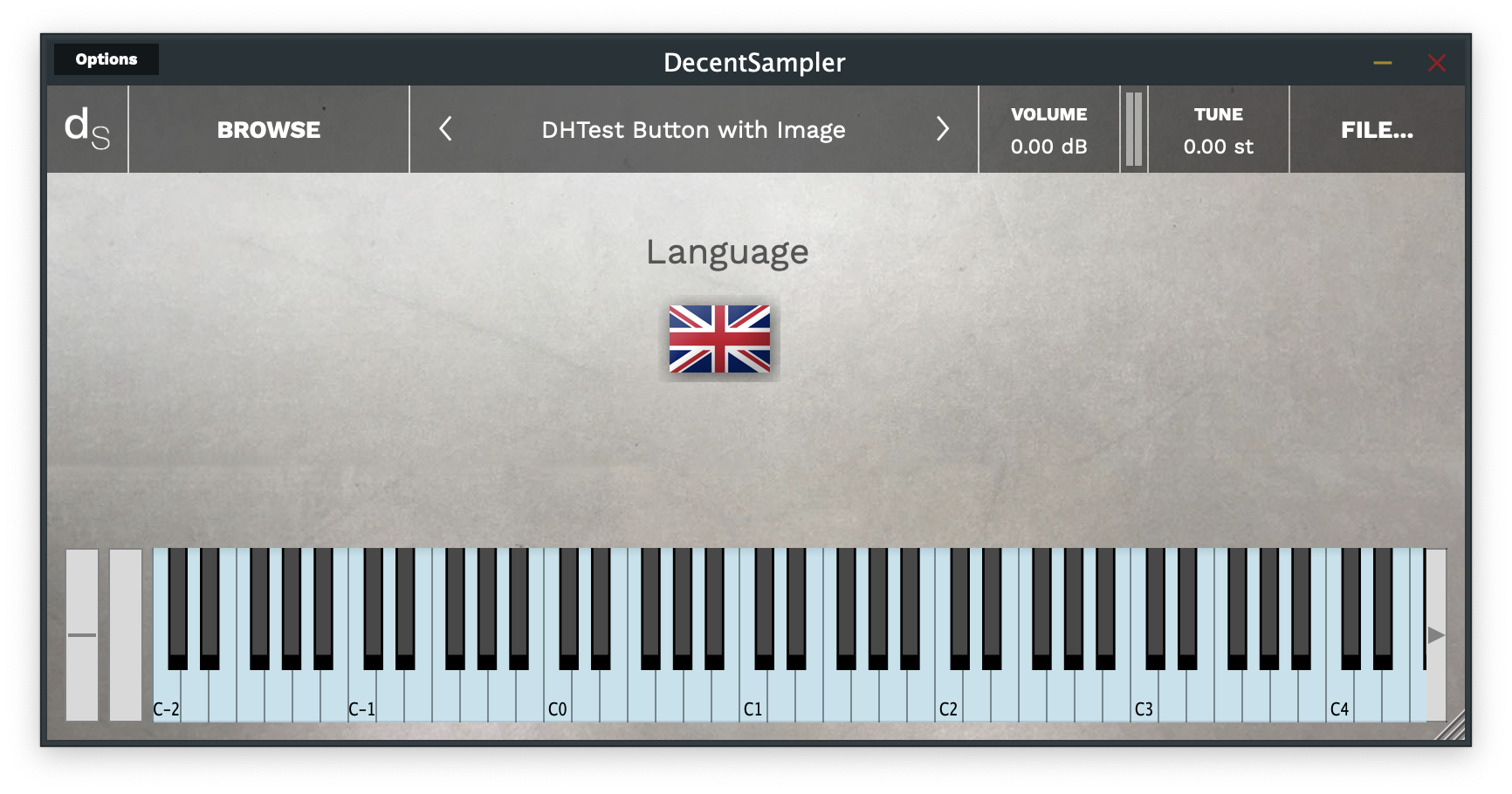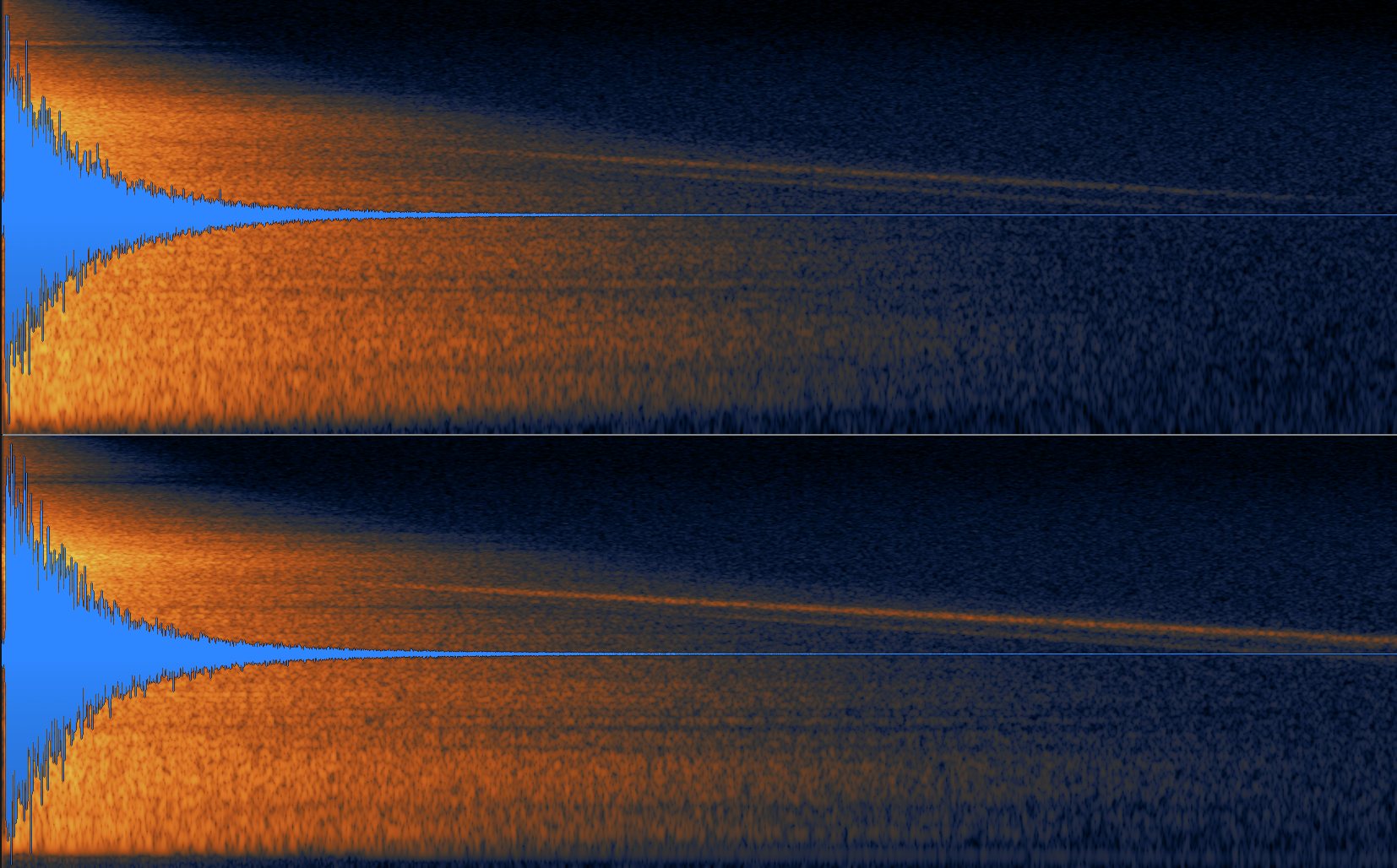Posted on

## For Sample Creators: How to create buttons in your sample librariesWell, it finally happened, version 1.7.0 of Decent Sampler introduces the concept of buttons to the world of Decent Sampler. To make a button in you user interface, simply use a <button> element:

```.wp-block-code {
border: 0;
}

.wp-block-code > span {
display: block;
overflow: auto;
}

.shcb-language {
border: 0;
clip: rect(1px, 1px, 1px, 1px);
-webkit-clip-path: inset(50%);
clip-path: inset(50%);
height: 1px;
margin: -1px;
overflow: hidden;
position: absolute;
width: 1px;
word-wrap: normal;
word-break: normal;
}

.hljs {
box-sizing: border-box;
}

.hljs.shcb-code-table {
display: table;
width: 100%;
}

.hljs.shcb-code-table > .shcb-loc {
color: inherit;
display: table-row;
width: 100%;
}

.hljs.shcb-code-table .shcb-loc > span {
display: table-cell;
}

.wp-block-code code.hljs:not(.shcb-wrap-lines) {
white-space: pre;
}

.wp-block-code code.hljs.shcb-wrap-lines {
white-space: pre-wrap;
}

.hljs.shcb-line-numbers {
border-spacing: 0;
counter-reset: line;
}

.hljs.shcb-line-numbers > .shcb-loc {
counter-increment: line;
}

.hljs.shcb-line-numbers .shcb-loc > span {
}

.hljs.shcb-line-numbers .shcb-loc::before {
border-right: 1px solid #ddd;
content: counter(line);
display: table-cell;
text-align: right;
-webkit-user-select: none;
-moz-user-select: none;
-ms-user-select: none;
user-select: none;
white-space: nowrap;
width: 1%;
}
```<button x="10" y="40"  width="120" height="30" style="image" value="0" mainImage="samples/ButtonMainImage.png" hoverImage="samples/ButtonHoverImage.png" clickImage="samples/ButtonSelectedImage.png">
<!-- Your button states go here. These are defined using the <state> element. -->
</button>
```Code language: HTML, XML (xml)```

There are two types of buttons: text and image. The value of the style attribute determines which kind of button gets created.

## Text Buttons

Text buttons are pretty basic, they look like this:

Here is the code for this button:

``````<button x="350" y="70"  width="120" height="40" style="text">
<state name="English">
<!-- Bindings go here -->
</state>
<state name="French">
<!-- Bindings go here -->
</state>
</button>
```Code language: HTML, XML (xml)```

As you can see, the actual text that gets displayed is defined in the name= parameter of each <state> element.

## Image Buttons

Now, let’s look at the image button. Here, you can use any image you want (even these ugly flag buttons I made in about 20 seconds in Photoshop):

The code for this button is as follows:

``````<button x="350" y="70"  width="70" height="50" style="image" value="0" >
<state name="English" mainImage="samples/EFlag_MainImage.png" hoverImage="samples/EFlag_HoverImage.png" clickImage="samples/EFlag_SelectedImage.png">
</state>
<state name="French" mainImage="Samples/FFlag_MainImage.png" hoverImage="Samples/FFlag_HoverImage.png" clickImage="Samples/FFlag_SelectedImage.png">
</state>
</button>
```Code language: HTML, XML (xml)```

As you can see, each <state> has three image parameters. Only the first one, mainImage, is required:

Bindings

Of course, if you want your buttons to actually do something, you’ll need to put <binding> elements underneath the <state> elements:

``````<button x="350" y="70"  width="120" height="40" style="text">
<state name="English">
<binding type="general" level="group" position="0" parameter="ENABLED" translation="fixed_value" translationValue="true" />
<binding type="general" level="group" position="1" parameter="ENABLED" translation="fixed_value" translationValue="false" />
</state>
<state name="French">
<binding type="general" level="group" position="1" parameter="ENABLED" translation="fixed_value" translationValue="true" />
<binding type="general" level="group" position="0" parameter="ENABLED" translation="fixed_value" translationValue="false" />
</state>
</button>
```Code language: HTML, XML (xml)```

As you can see, the example above uses a button to switch between two groups. You’ll note the liberal use of the `fixed_value`translation mode above. This means that when any of these options are selected, a fixed predetermined value is used for the value of that binding.

## Conclusion & Examples

OK. I think that’s it. You can download the examples used in this blog post here. If you find any bugs having to do with buttons, make sure you report them here.

Posted on

## For Sample Creators: How to use Convolution in your Decent Sampler presetsVersion 1.6.12 of Decent Sampler brings a Convolution effect to the Decent Sampler platform. If you don’t know what Convolution is, you can see a great explanation here. The most common use case for convolution is in creating reverb, and that is the use case that will be demonstrated here.

## How to add the Convolution effect to a preset

The convolution effect is invoked in much the same way that any other effect is defined:

``````<effects>
<effect type="convolution" mix="0.5" irFile="Samples/Hall_IR.wav" />
</effects>```Code language: HTML, XML (xml)```

As you can see, other than the required type attribute, there are two other attributes:

• The mix attribute controls how much of the convolved signal is present in the output. A value of 0 is completely dry whereas a value of 1 is completely wet containing only the convolved signal.
• The irFile attribute specifies the file that should be used as an impulse response or IR.

## How to control the convolution effect using UI controls

Two of the convolution effect’s attributes can be controlled using UI controls. The mix level can be controlled by a knob as follows:

``````<labeled-knob x="680" y="40" label="Conv Mix" type="float" minValue="0" maxValue="1" value="0.5" textColor="FF000000" >
<binding type="effect" level="instrument" position="0" parameter="FX_MIX" translation="linear"  />
</labeled-knob>```Code language: HTML, XML (xml)```

The IR impulse can be changed dynamically using a menu control:

``````<label text="IR File" x="480" y="40" width="120" height="30"></label>
<menu x="580" y="40"  width="120" height="30" requireSelection="true" placeholderText="Choose..." value="1">
<option name="long hall.wav">
<binding type="effect" level="instrument" position="1" parameter="FX_IR_FILE" translation="fixed_value" translationValue="Samples/long hall.wav" />
</option>
<option name="ABLCR Chord Vocal.aif">
<binding type="effect" level="instrument" position="1" parameter="FX_IR_FILE" translation="fixed_value" translationValue="Samples/ABLCR Chord Vocal.aif" />
</option>
<option name="Amp Spring High.aif">
<binding type="effect" level="instrument" position="1" parameter="FX_IR_FILE" translation="fixed_value" translationValue="Samples/Amp Spring High.aif" />
</option>
<option name="Swede Plate 3.5s.aif">
<binding type="effect" level="instrument" position="1" parameter="FX_IR_FILE" translation="fixed_value" translationValue="Samples/Swede Plate 3.5s.aif" />
</option>